# Riemann Sum Program for the TI-83+ and TI-84+

Filed under Calculus, Difficulty: Easy, TI-83 Plus, TI-84 Plus.

RIEMANN, a program for the TI-83+ and TI-84+, approximates the area under a curve (integral) by calculating a Riemann sum, a sum of areas of simple geometric figures intersecting the curve. The program solves Riemann sums using one of four methods and displays a graph when prompted. The program itself is optimized to smaller than 1 kilobyte to reduce its memory impact. This tutorial covers the theory of Riemann sums and how to use this program.

## Theory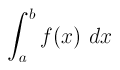This definite integral represents the net area between a function and the x axis between x=a and x=b.

Integrals are used in the natural and social sciences, statistics, economics, finance, and more, but oftentimes they are difficult to calculate. One method of calculating integrals is to look at the basic definition of an integral, as the area underneath a curve.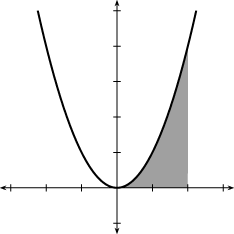Visual representation of an integral under the curve y=x²

We can approximate this area by taking a series of rectangles and packing them underneath the curve. Unlike a smooth curve, it is easy to calculate the area of a rectangle, (base*height), and the more, smaller rectangles we use, the more accurate the approximation will be. There are actually many different ways of placing rectangles to choose from, and using trapezoids is an even more effective approach, but all of these sums converge to the actual integral. Below are 3 different visual representations of Riemann sums: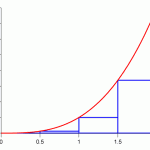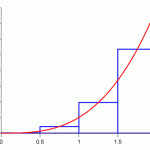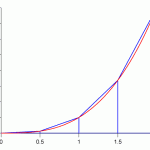Left, Middle, and Trapezoid Riemann Sums

## Using the RIEMANN Program

Download RIEMANN.8xp and send the file to your calculator. If you’re not familiar with this process, you can view a tutorial here. To begin, enter the equation you want to use for calculations in the Y1 variable. The Y-Variables screen can be reached by pressing . If your screen doesn’t look like this, press and make sure “Func” is selected.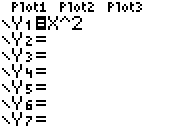Y= Variable Screen

Press , select RIEMANN, and press to run.Program on Home Screen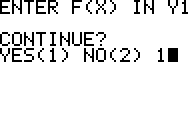If you have your function in Y1, enter 1 to continue.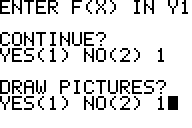Enter 1 to draw a graph, or 2 to just solve numerically.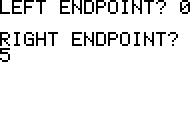Enter the numbers for the left and right endpoints of the Integral.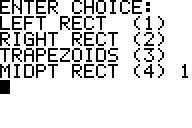Enter the number corresponding to the method you want to use.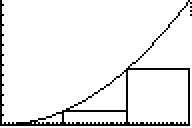The resulting graph.

Press to continue.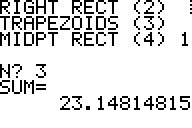The numeric result corresponding to the area of the intervals.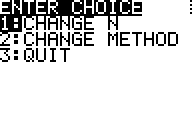You can choose to change the settings for your calculations or exit the program.

When a program is not in use, it is generally a good idea to archive it in order to conserve RAM.

```PRGM:RIEMANN ClrHome PlotsOff Disp "ENTER F(X) IN Y1 Disp " Disp "CONTINUE? Input "YES(1) NO(2) ",G If G=2:Stop Disp " Disp "DRAW PICTURES? Input "YES(1) NO(2) ",H ClrHome Input "LEFT ENDPOINT? ",A Input "RIGHT ENDPOINT? ",B If H=1:Then A->Xmin:B->Xmax iPart(((B-A)/20->W If W=0:Goto Z Lbl U seq(X,X,A,B,W->L5 Y1(L5->L6 min(L6->Ymin If Ymin>0:0->Ymin max(L6->Ymax If Ymax<0:0->Ymax W->Xscl iPart((abs((Ymax-Ymin)/10->Yscl ClrList L6,L5 End Lbl 0 ClrHome Disp "ENTER CHOICE: Disp "LEFT RECT (1) Disp "RIGHT RECT (2) Disp "TRAPEZOIDS (3) Input "MIDPT RECT (4) ",R Lbl 1 ClrDraw Input "N? ",N (B-A)/N->W 0->S:1->C Lbl 2 If R=1:Goto 3 If R=2:Goto 4 If R=3:Goto 3 If R=4:Goto 5 Lbl 3 A+W(C-1->X X->J:X+W->L Goto 7 Lbl 4 A+CW->X X-W->J:X->L Goto 7 Lbl 5 If H≠1:Then If N>5:Then 1->Z:W/2->H:A->X Lbl 8 X+H->X:Y1+S->S A+ZW->X IS>(Z,N:Goto 8 SW->S:Goto T End:End A+CW-W/2->X X-W/2->J X+W/2->L Goto 7 A->G:G+W->G:G->V Lbl 9 V->X:Y1->Y:V+W->X:4Y+2Y1+S->S V+2W->V If V G-W->X:Y1->E B->X:Y1->F (W/3)(S+E-F->S Goto T Lbl 7 Y1->K:K+S->S If H=1:Goto D Lbl I IS>(C,N Goto 2 If R=3:Then A->X:Y1->P B->X:Y1->Q S+(Q-P)/2->S End W*S->S Lbl T Disp "SUM=",S Pause ClrHome Lbl E Menu("ENTER CHOICE","CHANGE N",1,"CHANGE METHOD",0,"QUIT",F) Lbl F Stop Lbl D If R=3:Then Y1(L->M Else:K->M End Line(J,0,J,K Line(J,K,L,M Line(L,M,L,0 If C=N:Pause Goto I Lbl Z If B-A≤9:Then .1->W:Else 1->W:End Goto U ```

### 6 Responses to Riemann Sum Program for the TI-83+ and TI-84+

1.R. Dodds

What does the Y mean? I can’t get the program to work without knowing what it is.

•calcblog

There was a typo in the code–fixed it! Y1 refers to the graph variable which can be reached be pressing from the programming menu. It’s probably easier to just download this program to your calculator. (There’s a link in the downloads section.)2.Anonymous

When I tried to run this program, my calculator gave me an error syntax message which it located at lines:
IS>(Z,N:Goto 8
IS>(C,N
It also gave me a window range error when I attempted to get and type of rectangle on my graph and brought me to the line:
Line(J,0,J,K
I took out the two syntax error program steps that I listed above and then attempted to run the program again. When I tried the graphing option, the graph would only calculate and show one rectangle before the calculating indicator just kept running without anything happening. When I broke from this and hit the go to option the calculator took me to the step:
Lbl D
When I tried the non graphing option which would just calculate the sum, the same thing happened where the calculating indicator kept running after I had entered the endpoints, what I wanted to calculate, and n. This time the calculator took me to the step:
Lbl 7
I don’t have extensive knowledge in programming or understand the concept of all the different tedious steps of the program. I also don’t really have a clue how to fix these errors which is why I need some assistance. I copied the code on this web page verbatim into my calculator and the only theory I have for why it doesn’t work is because somewhere along the line I have confused the letter I with the number 1, (or maybe the letter O with the number 0 but I don’t think the letter O was used as a variable in this code). My only attempt at a solution was taking out the syntax error lines I have listed above but that didn’t work as the calculator wasn’t able to complete calculations for either the sum or the rectangles in the graph. This is the best and most detailed and useful program I have found online and if I were able to get it to work I could save loads of time on my Calculus Homework. I hope you will be able to help me solve the problem I am facing.

3.Dave

I can’t seem to find the “Xmin”, “Ymin”, “Xmax”, or “Ymin” options on my calculator, unless it means the values assigned to them in WINDOW.

•Eric

They are found under vars 1 (i.e. vars window).

4.Eric

When I am using the program, it doesn’t allow me to choose which time of Riemann sum I want to use (i.e. left, right, trapezoid, or midpoint). Rather, it shows on the line below those options “544” and below that line it gives me the option to choose my N. When I’ve tried testing it out with different numbers and equations, every time it only gives me the left side Riemann sum, allowing me to change only my N and not my “method.” I have tried looking at my code and the published code to look for any discrepancies but haven’t found any to my knowledge. If you could help me with this for my Calculus class as soon as possible, it would be very much appreciated. Thank you!